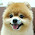## 2013年5月13日 星期一

### List.aspx 使用 Sample.Repository.ADONET

List.aspx 的 Code Behind 完整程式如下：

`using System;`
`using System.Collections.Generic;`
`using System.Linq;`
`using System.Web;`
`using System.Web.UI;`
`using System.Web.UI.WebControls;`
`using Sample.Repository.Interface;`
`using Sample.Repository.ADONET;`
` `
`namespace Sample.Web.WebForm.Employee`
`{`
`    public partial class List : System.Web.UI.Page`
`    {`
`        private IEmployeeRepository _repository;`
` `
`        public List()`
`        {`
`            this._repository = new EmployeeRepository();`
`        }`
` `
` `
`        protected void Page_Load(object sender, EventArgs e)`
`        {`
`            if (!this.IsPostBack)`
`            {`
`                this.EnployeesDataBind();`
`            }`
`        }`
` `
`        private void EnployeesDataBind()`
`        {`
`            var employees = this._repository.GetEmployees();`
`            this.GridView1.DataSource = employees;`
`            this.GridView1.DataKeyNames = new string[] { "EmployeeID" };`
`            this.GridView1.DataBind();`
`        }`
` `
`        protected void GridView1_RowDataBound(object sender, GridViewRowEventArgs e)`
`        {`
`            if (e.Row.RowType == DataControlRowType.DataRow)`
`            {`
`                object primaryKey = GridView1.DataKeys[e.Row.RowIndex]["EmployeeID"];`
`                HyperLink HyperLink_Details = e.Row.FindControl("HyperLink_Details") as HyperLink;`
` `
`                int employeeID;`
`                if (int.TryParse(primaryKey.ToString(), out employeeID)`
`                    && HyperLink_Details != null)`
`                {`
`                    HyperLink_Details.NavigateUrl = string.Concat("Details.aspx?id=", employeeID.ToString());`
`                }`
`            }`
`        }`
` `
`    }`
`}`

### Details.asp 使用 Sample.Repository.ADONET

Code Behind 的程式內容：

`using System;`
`using System.Collections.Generic;`
`using System.Linq;`
`using System.Web;`
`using System.Web.UI;`
`using System.Web.UI.WebControls;`
`using Sample.Repository.Interface;`
`using Sample.Repository.ADONET;`
` `
`namespace Sample.Web.WebForm.Employee`
`{`
`    public partial class Details : System.Web.UI.Page`
`    {`
`        private IEmployeeRepository _repository;`
` `
`        public Details()`
`        {`
`            this._repository = new EmployeeRepository();`
`        }`
` `
`        private int employeeID;`
`        public int EmployeeID`
`        {`
`            get { return employeeID; }`
`            set { employeeID = value; }`
`        }`
` `
`        private Sample.Domain.Employee instance;`
`        public Sample.Domain.Employee Instance`
`        {`
`            get`
`            {`
`                if (this.instance == null)`
`                {`
`                    var employee = this._repository.GetOne(this.EmployeeID);`
`                    this.instance = employee;`
`                }`
`                return instance;`
`            }`
`        }`
` `
`        protected void Page_Load(object sender, EventArgs e)`
`        {`
`            if (!Page.IsPostBack)`
`            {`
`                this.SetDefault();`
`            }`
`        }`
` `
`        private void SetDefault()`
`        {`
`            int id;`
`            if (Request.QueryString["id"] == null)`
`            {`
`                Response.Redirect("List.aspx");`
`            }`
`            else`
`            {`
`                if (!int.TryParse(Request.QueryString["id"].Trim(), out id))`
`                {`
`                    Response.Redirect("List.aspx");`
`                }`
`                else`
`                {`
`                    this.EmployeeID = id;`
`                    if (this.Instance == null)`
`                    {`
`                        Response.Redirect("List.aspx");`
`                    }`
`                    else`
`                    {`
`                        this.Label_EmployeeID.Text = this.Instance.EmployeeID.ToString();`
`                        this.TextBox_LastName.Text = this.Instance.LastName;`
`                        this.TextBox_FirstName.Text = this.Instance.FirstName;`
`                        this.TextBox_Title.Text = this.Instance.Title;`
`                        this.TextBox_BirthDate.Text = this.Instance.BirthDate.ToString("yyyy/MM/dd");`
`                        this.TextBox_HireDate.Text = this.Instance.HireDate.ToString("yyyy/MM/dd");`
`                    }`
`                }`
`            }`
`        }`
`    }`
`}`

Details.aspx 執行結果

### 相關系列文章

ASP.NET MVC

ASP.NET WebForm

ASP.NET MVC 與 ASP.NET WebForm 使用 Simple Injector 切換選擇不同 Repository 原始碼下載

#### 2 則留言:

1.謝謝版主分享 ^ ^.

1.希望這樣的說明可以讓你有一些些的幫助。